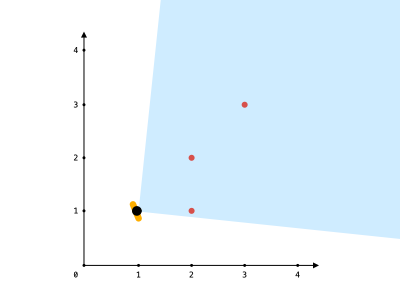# Leetcode 1610 - Maximum Number of Visible Points

Note:

• How to calculate polar angle? - The angle between a point and the origin. ([-PI, PI]).
•• Use Math.atan2(x, y) in JS. The result is in rad.
• For every point other that the location, calculate its polar angle and put it into angles[].
• Sort them in ascending order.
• A tricky detail:
•• The diff between these two angles are actually < angle. But because a > 0 and b < 0, the result from atan2(a, b) might > angle.
• How to solve this?
• Add 360 to each angle after sorting, and append the array to angles[].
• Assume b was in the very front, and a was the biggest in the end.
• After appending the new array, now a and b + 360 are neighbors!
• Something like [b, ..., a, b + 360, ..., a + 360].
• Use sliding window to find the longest length when angles[r] - angles[l] <= angle.

Question:

You are given an array points, an integer angle, and your location, where location = [posx, posy] and points[i] = [xi, yi] both denote integral coordinates on the X-Y plane.

Initially, you are facing directly east from your position. You cannot move from your position, but you can rotate. In other words, posx and posy cannot be changed. Your field of view in degrees is represented by angle, determining how wide you can see from any given view direction. Let d be the amount in degrees that you rotate counterclockwise. Then, your field of view is the inclusive range of angles [d - angle/2, d + angle/2].

You can see some set of points if, for each point, the angle formed by the point, your position, and the immediate east direction from your position is in your field of view.

There can be multiple points at one coordinate. There may be points at your location, and you can always see these points regardless of your rotation. Points do not obstruct your vision to other points.

Return the maximum number of points you can see.

Example:Code: